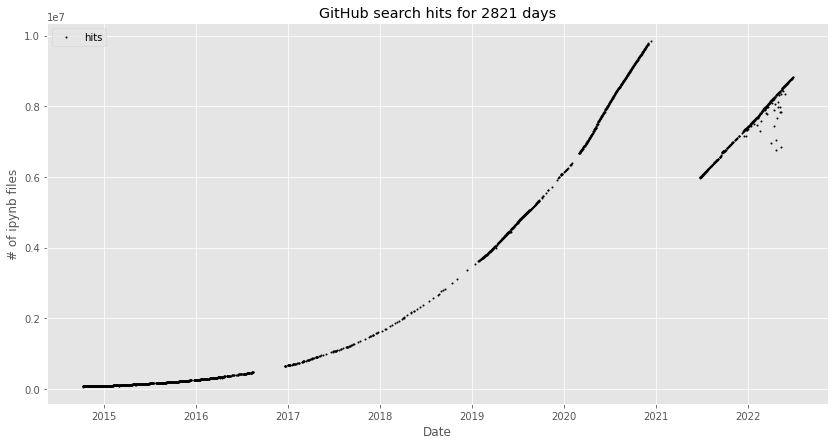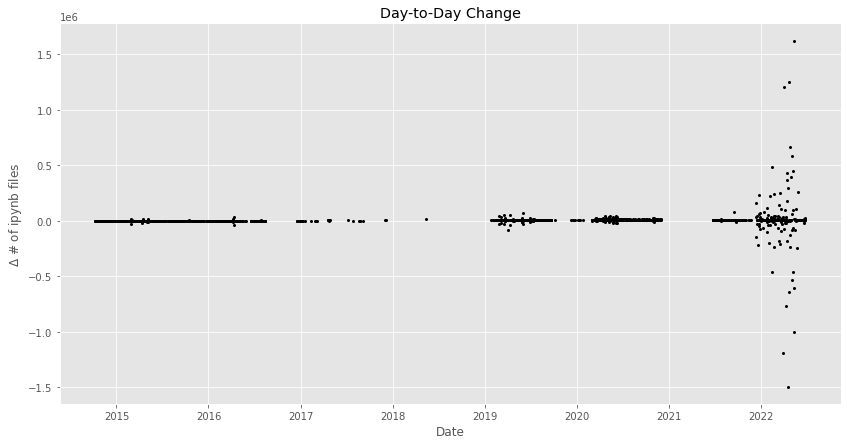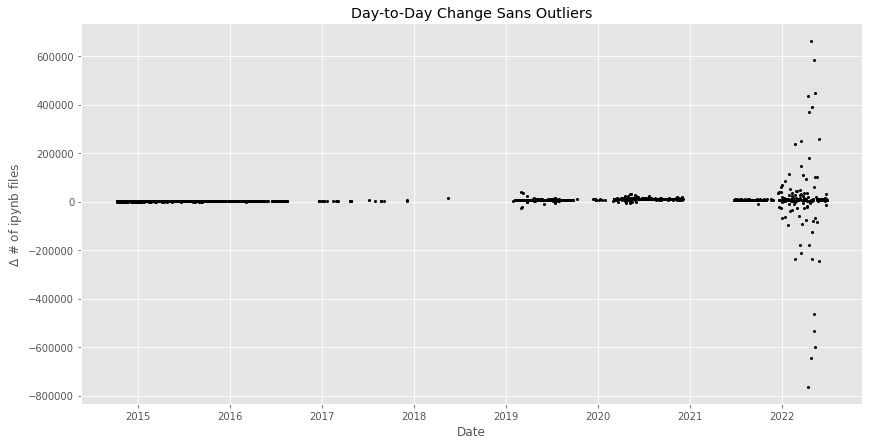In :
from IPython.display import Markdown

Out:

# Estimate of Public Jupyter Notebooks on GitHub¶

## Data Collection History¶

• Late-2014 to mid-2016: I wrote a script that scrapes the GitHub web search UI for the count, appends to a CSV, executes a notebook, and stores the results in a gist at https://gist.github.com/parente/facb555dfbae28e817e0. I scheduled the script to run daily.
• Mid-2106 to Late-2016: The GitHub web search UI started requiring authentication to see global search results. I stopped collecting data.
• Late-2016 to early-2019: I rewrote the process to include a human-in-the-loop who entered the hit count after viewing the search results page. I moved the CSV, notebook, and scripts to this repo, and sporadically ran the script.
• Early-2019: I found out that the GitHub search API now supports global search. I automated the entire collection process again and set it to run on TravisCI on a daily schedule.
• December 2020: GitHub changed their code search index results to exclude repositories without activity for the past year. The ipynb search result count dropped from nearly 10 million to 4.5 million ipynb files, stayed there for a day or so, and then began climbing again from that new origin.
• June 2021: I started collecting data again but disabled the notebook showing the historical and predicted counts.
• July 2021: I revived the notebook showing the historical counts but kept prediction disabled.

## Assumptions¶

• That the search query hits are less than or equal to the total number of *.ipynb files on GitHub.
• That the result is not inflated due to GitHub forks.
• Evidence: We do not see the tutorial notebooks from the ipython/ipython GitHub repository duplicated in the search results because of the 2,000+ forks of the ipython/ipython repo.
• That the result is inflated a tiny bit by manually created duplicates of notebooks.
• Evidence: Some people seem to download their favorite notebooks and then upload them into their own git repositories for safe keeping.
In :
%matplotlib inline

In :
import datetime
import matplotlib as mpl
import matplotlib.pyplot as plt
import numpy as np
import pandas as pd
import requests

In :
mpl.style.use('ggplot')
figsize = (14,7)

In :
now = datetime.datetime.utcnow()
print(f'This notebook was last rendered at {now} UTC')

This notebook was last rendered at 2022-06-27 05:16:41.360950 UTC


First, let's load the historical data into a DataFrame indexed by date.

In :
hits_df = pd.read_csv('ipynb_counts.csv', index_col=0, header=0, parse_dates=True)
hits_df.reset_index(inplace=True)
hits_df.drop_duplicates(subset='date', inplace=True)
hits_df.set_index('date', inplace=True)
hits_df.sort_index(ascending=True, inplace=True)

In :
hits_df.tail(3)

Out:
hits
date
2022-06-25 8808305
2022-06-26 8813359
2022-06-27 8818675

There might be missing counts for days that we failed to sample. We build up the expected date range and insert NaNs for dates we missed.

In :
til_today = pd.date_range(hits_df.index, hits_df.index[-1])

In :
hits_df = hits_df.reindex(til_today)


Now we plot the known notebook counts.

In :
fig, ax = plt.subplots(figsize=figsize)
ax.set_title(f'GitHub search hits for {len(hits_df)} days')
ax.plot(hits_df.hits, 'ko', markersize=1, label='hits')
ax.legend(loc='upper left')
ax.set_xlabel('Date')
ax.set_ylabel('# of ipynb files');Growth appears exponential until December 2020, at which point the count dropped suddenly and resumed growth from a new origin.

The total change in the number of *.ipynb hits between the first day we have data and today is:

In :
total_delta_nbs = hits_df.iloc[-1] - hits_df.iloc
total_delta_nbs

Out:
hits    8752827.0
dtype: float64

The mean daily change for the entire duration is:

In :
avg_delta_nbs = total_delta_nbs / len(hits_df)
avg_delta_nbs

Out:
hits    3102.7391
dtype: float64

The change in hit count between any two consecutive days for which we have data looks like the following:

In :
daily_deltas = (hits_df.hits - hits_df.hits.shift())

In :
fig, ax = plt.subplots(figsize=figsize)
ax.plot(daily_deltas, 'ko', markersize=2)
ax.set_xlabel('Date')
ax.set_ylabel('$\Delta$ # of ipynb files')
ax.set_title('Day-to-Day Change');The large jumps in the data are from GitHub reporting drastically different counts from one day to the next.

Let's drop outliers defined as values more than two standard deviations away from a centered 90 day rolling mean.

In :
daily_delta_rolling = daily_deltas.rolling(window=90, min_periods=0, center=True)
outliers = abs(daily_deltas - daily_delta_rolling.mean()) > 2*daily_delta_rolling.std()
outliers.value_counts()

Out:
False    2751
True       70
Name: hits, dtype: int64
In :
cleaned_hits_df = hits_df.copy()
cleaned_hits_df[outliers] = np.NaN
cleaned_daily_deltas = (cleaned_hits_df.hits - cleaned_hits_df.hits.shift())

In :
fig, ax = plt.subplots(figsize=figsize)
ax.plot(cleaned_daily_deltas, 'ko', markersize=2)
ax.set_xlabel('Date')
ax.set_ylabel('$\Delta$ # of ipynb files')
ax.set_title('Day-to-Day Change Sans Outliers');